# RD Sharma Class 9 Solutions Chapter 2 Exponents of Real Numbers MCQS

## RD Sharma Class 9 Solutions Chapter 2 Exponents of Real Numbers MCQS

These Solutions are part of RD Sharma Class 9 Solutions. Here we have given RD Sharma Class 9 Solutions Chapter 2 Exponents of Real Numbers MCQS

Mark the correct alternative in each of the following:
Question 1.
The value of {2 – 3 (2 – 3)3}3 is
(a) 5
(b) 125
(c) $$\frac { 1 }{ 5 }$$
(d) -125
Solution:
{2 – 3 (2 – 3)3}3 = {2 – 3 (-1)3}3
= {2 – 3 x (-1)}3
= (2 + 3)3 = (5)3
= 125    (b)

Question 2.
The value of x – yx-y when x = 2 and y = -2 is
(a) 18
(b) -18
(c) 14
(d) -14
Solution:
x = 2, y = -2
x-yx-y = 2 – (-2)2 – (-2)
= 2 – (-2)2 + 2 = 2 – (-2)4
= 2 – (+16) = 2 – 16 = -14        (d)

Question 3.
The product of the square root of x with the cube root of x, is
(a) cube root of the square root of x
(b) sixth root of the fifth power of x
(c) fifth root of the sixth power of x
(d) sixth root of x
Solution: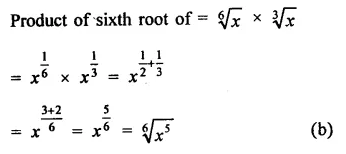Question 4.
The seventh root of x divided by the eighth root of x is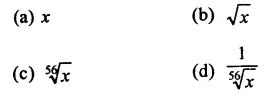Solution: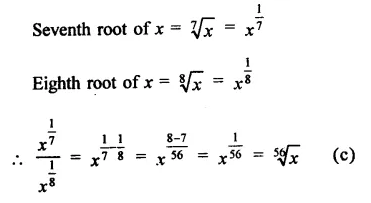Question 5.
The square root of 64 divided by the cube root of 64 is
(a) 64
(b) 2
(c) $$\frac { 1 }{ 2 }$$
(d) 64$$\frac { 2 }{ 3 }$$
Solution: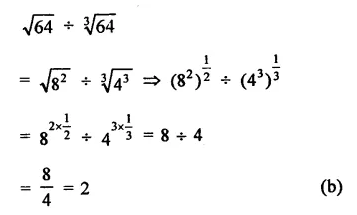Question 6.
Which of the following is (are) not equal to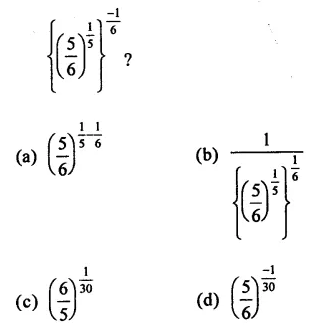Solution: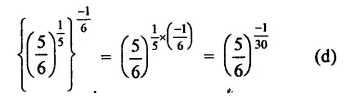Question 7.
When simplified (x1 + y1)1 is equal to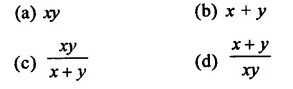Solution: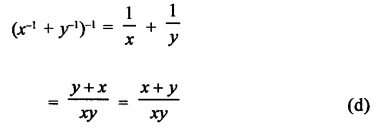Question 8.
If 8x+1 = 64, what is the value of 3 2x +1?
(a) 1
(b) 3
(c) 9
(d) 27
Solution: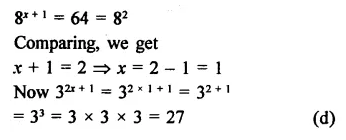Question 9.
If (23)2 = 4x then   3x =
(a) 3
(b) 6
(c) 9
(d) 27
Solution: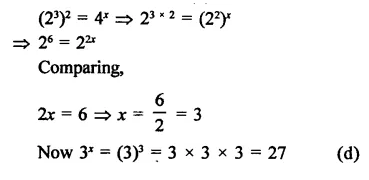Question 10.
If x-2= 64, then x$$\frac { 1 }{ 3 }$$ + x°=
(a) 2
(b) 3
(c) $$\frac { 3 }{ 2 }$$
(c) $$\frac { 2 }{ 3 }$$
Solution: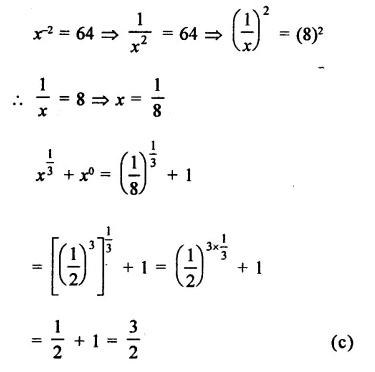Question 11.
When simplified ( –$$\frac { 1 }{ 27 }$$)$$\frac { -2 }{ 3 }$$
(a) 9
(b) -9
(c) $$\frac { 1 }{ 9 }$$
(d) –$$\frac { 1 }{ 9 }$$
Solution: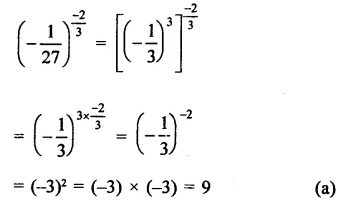Question 12.
Which one of the following is not equal to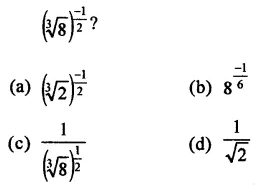Solution: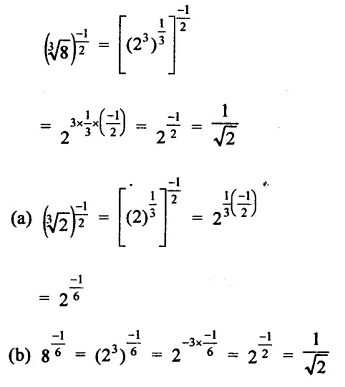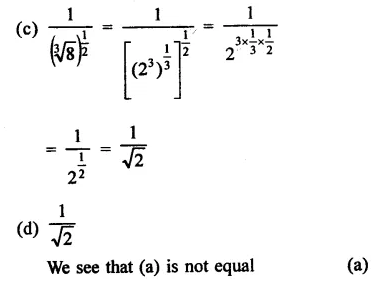Question 13.
Which one of the following is not equal to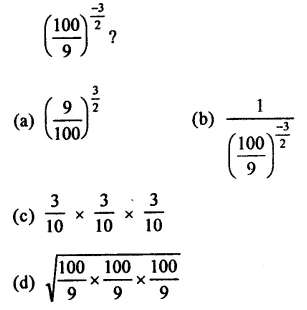Solution: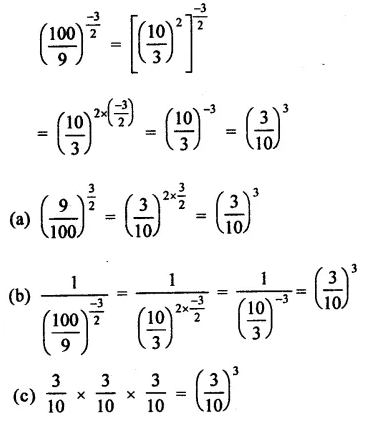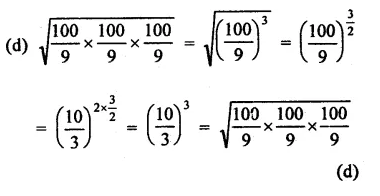Question 14.
If a, b, c are positive real numbers, then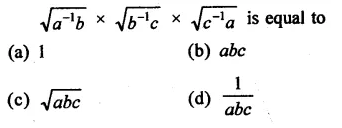Solution: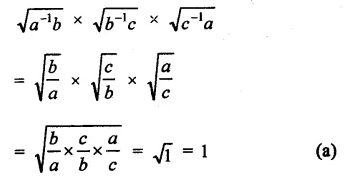Question 15.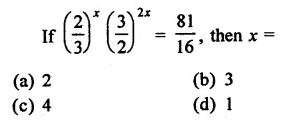Solution: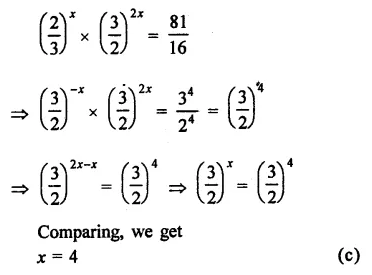Question 16.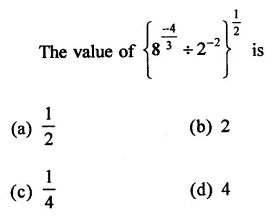Solution: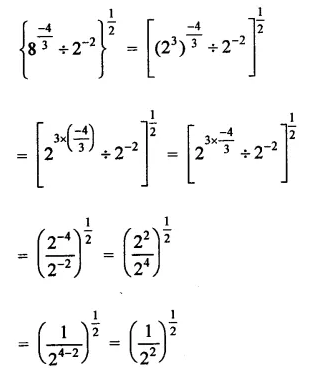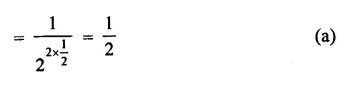Question 17.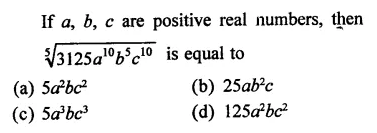Solution: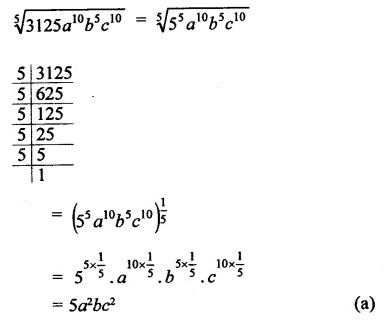Question 18.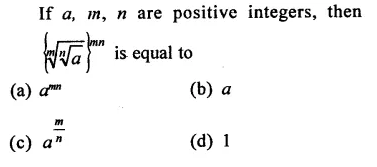Solution: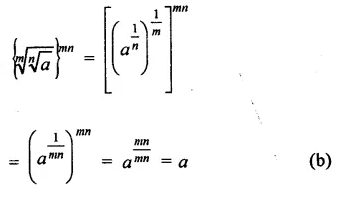Question 19.Solution: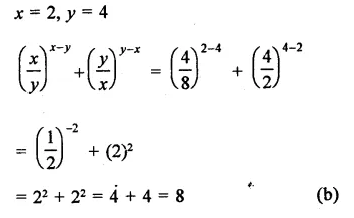Question 20.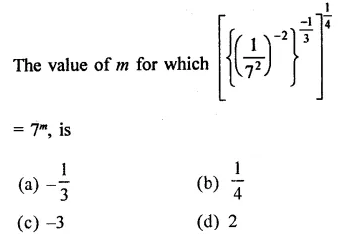Solution: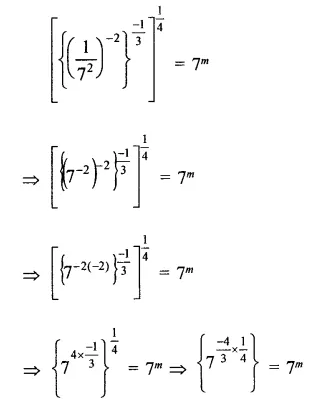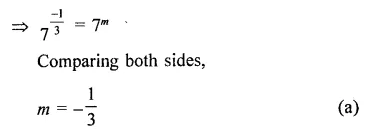Question 21.
The value of {(23 + 22)2/3+ (150 -29)1/2}2  is
(a) 196
(b) 289
(c) 324
(d) 400
Solution:
{(23 + 22)2/3 + (150 – 29)1/2}2
= [(23×4)$$\frac { 2 }{ 3 }$$  +(150 – 29)$$\frac { 1 }{ 2 }$$ ]2
= [(27)$$\frac { 2 }{ 3 }$$ + (121)$$\frac { 1 }{ 2 }$$ ]2
= [(33)3 +(112)$$\frac { 1 }{ 2 }$$]2 = (9 + 11)2
= (20)2 = 400  (d)

Question 22.
(256)0.16x (256)0.09
(a) 4
(b) 16
(c) 64
(d) 256.25
Solution: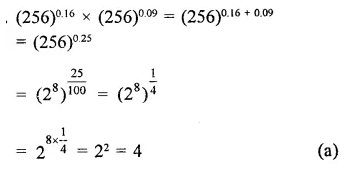Question 23.
If 102y = 25, then 10-y equals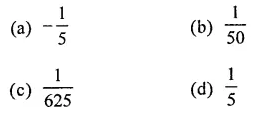Solution: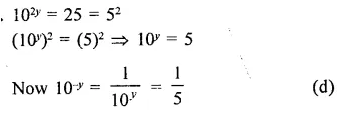Question 24.
If 9X + 2 = 240 + 9X. then x =
(a) 0.5
(b) 0.2
(c) 0.4
(d) 0.1
Solution: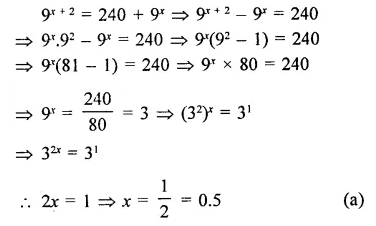Question 25.
If x is a positive real number and x2 = 2, then x3 =
(a) $$\sqrt { 2 }$$
(b) 2$$\sqrt { 2 }$$
(c) 3$$\sqrt { 2 }$$
(d) 4
Solution: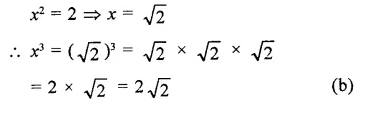Question 26.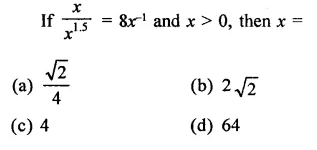Solution: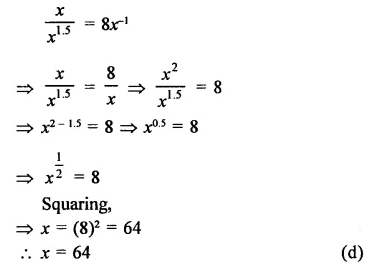Question 27.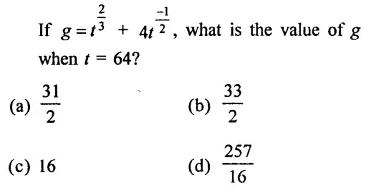Solution: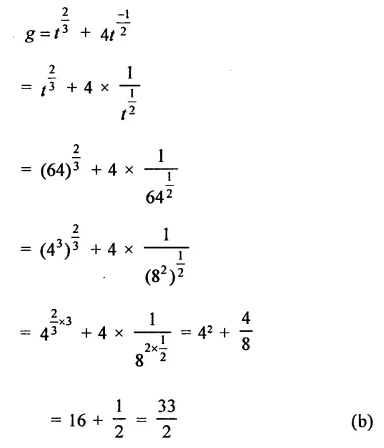Question 28.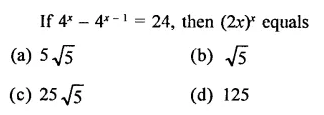Solution: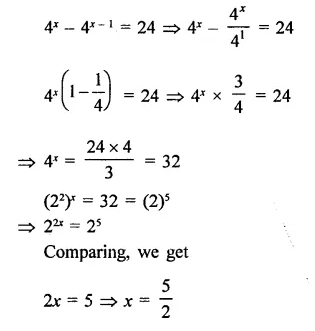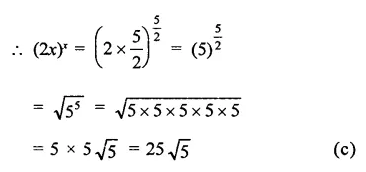Question 29.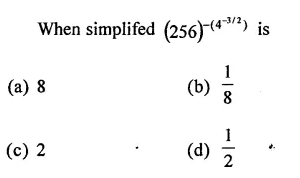Solution: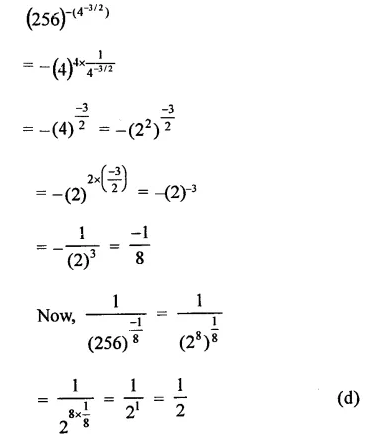Question 30.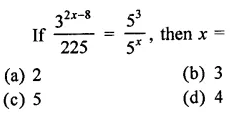Solution: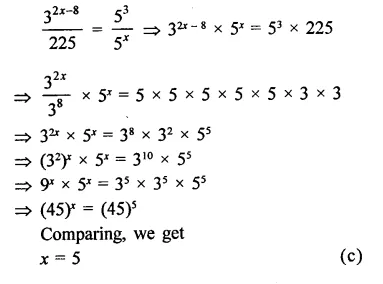Question 31.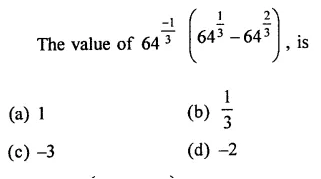Solution: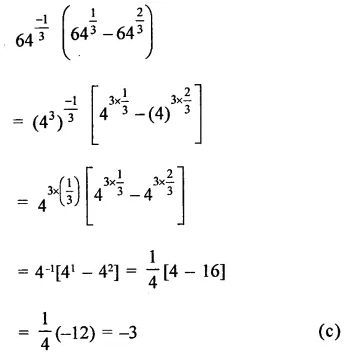Question 32.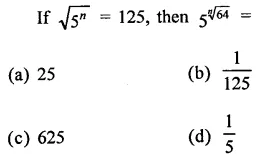Solution: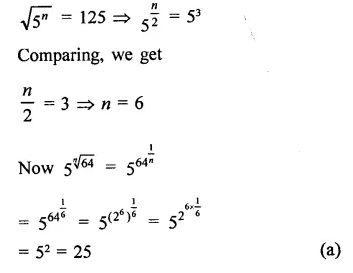Question 33.
If (16)2x + 3 = (64)x + 3 , then 42x – 2  =
(a) 64
(b) 256
(c) 32
(d) 512
Solution: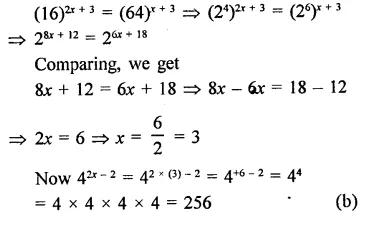Question 34.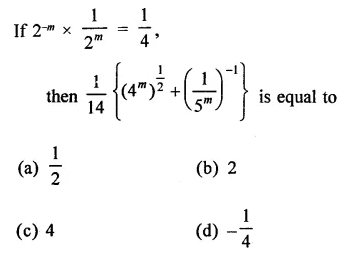Solution: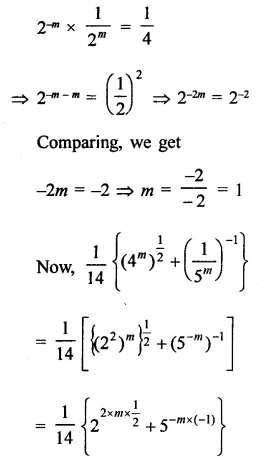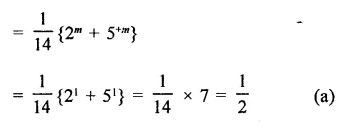Question 35.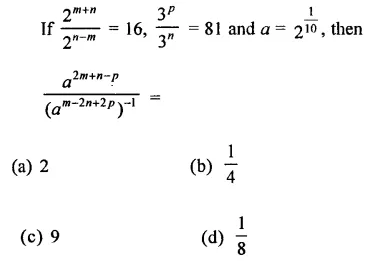Solution: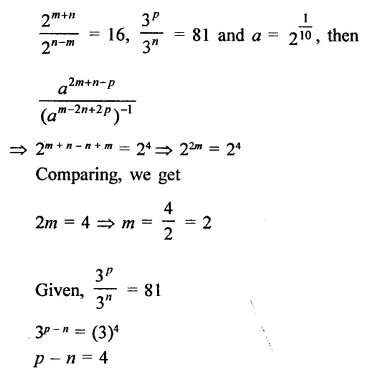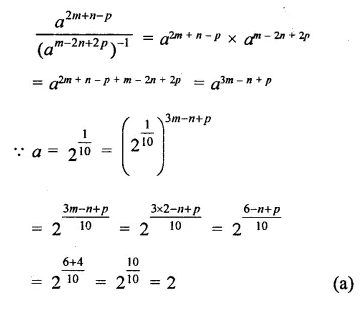Question 36.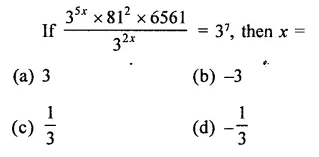Solution: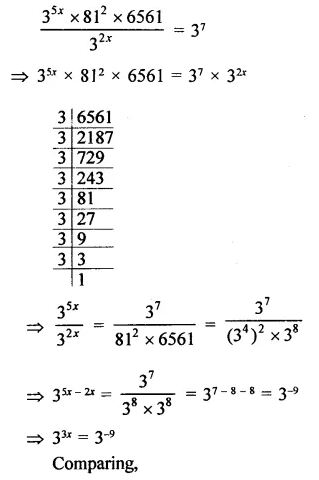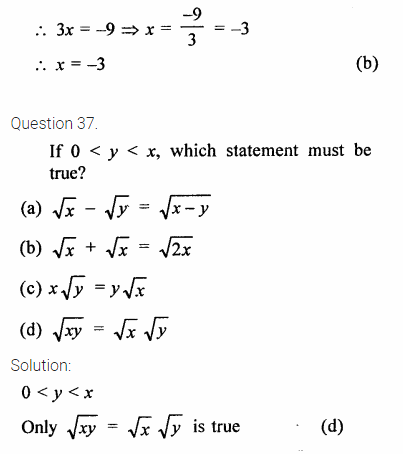Question 37.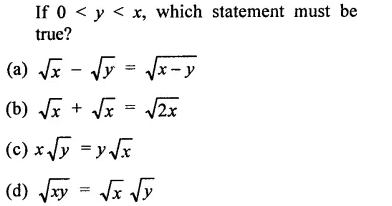Solution: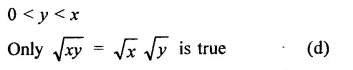Question 38.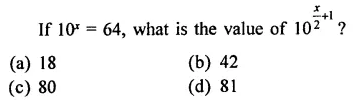Solution: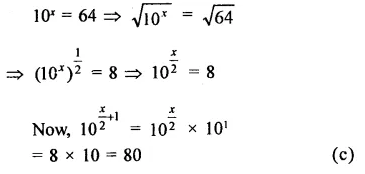Question 39.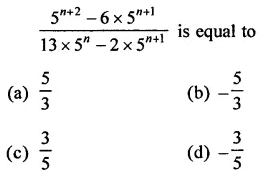Solution: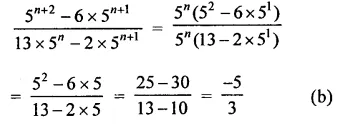Question 40.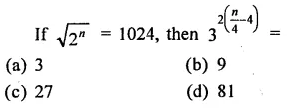Solution: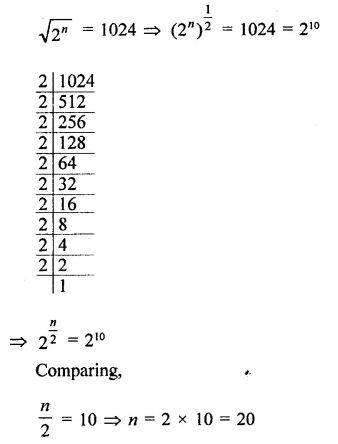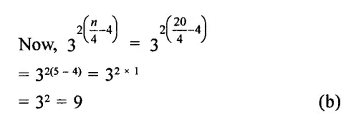Hope given RD Sharma Class 9 Solutions Chapter 2 Exponents of Real Numbers MCQS are helpful to complete your math homework.

If you have any doubts, please comment below. Learn Insta try to provide online math tutoring for you.